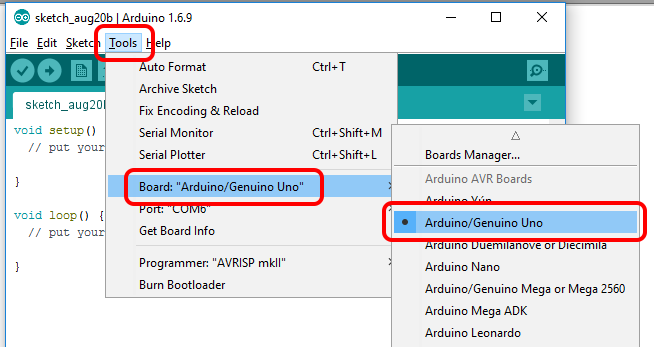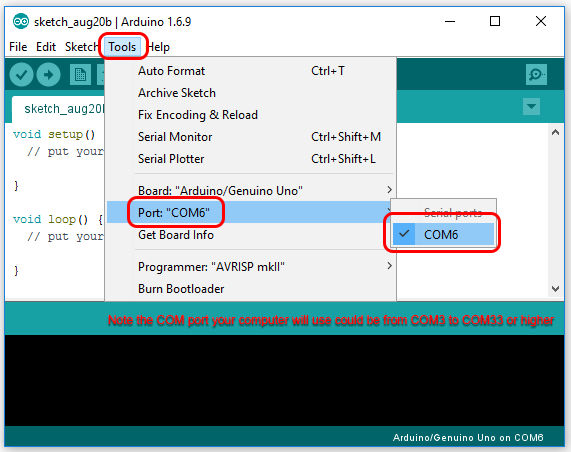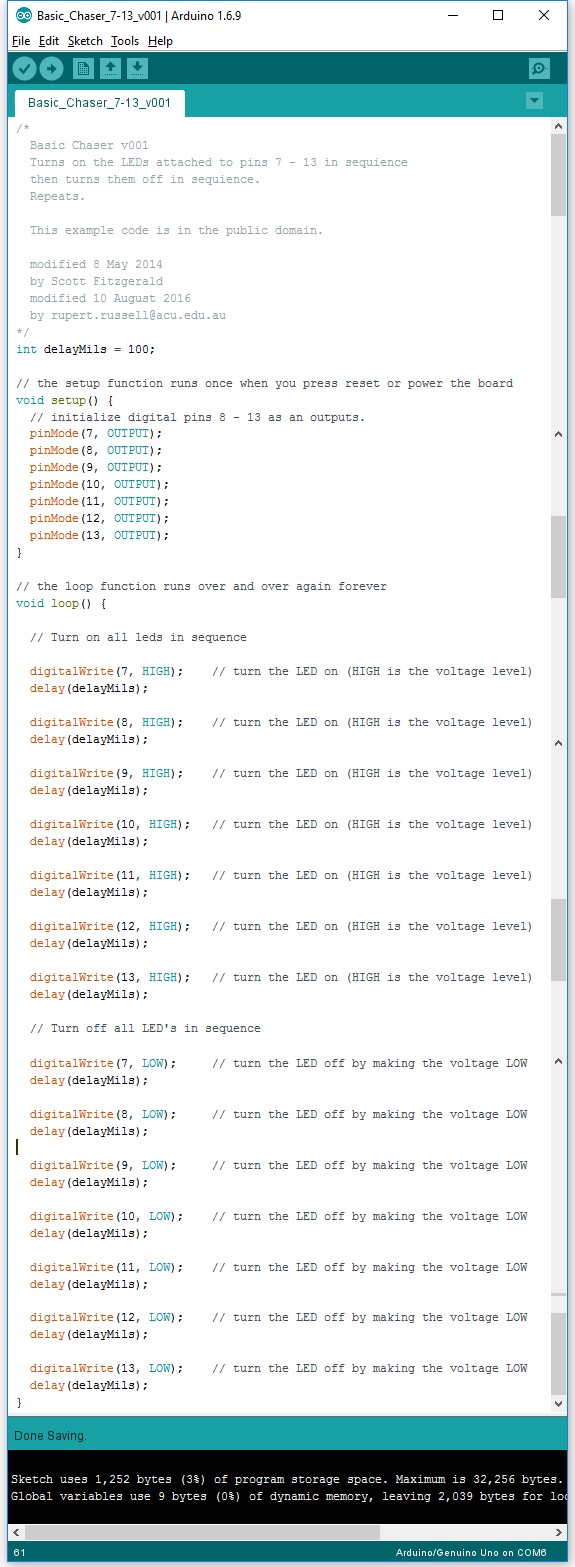Home > Tutorials > Auduino > Arduino UNO Demo > Basic Chaser
Home > COMM140 > Basic Chaser

# Basic Chaser

2) Connect the Arduino to the computer

4) Select the correct board type within the IDE for this example I am using a KnockOffDuino Uno compatiable board.5) Identify and select the correct COM port6) Create a new sketch and copyu and paste the example Basic Chaser code below

 /*   Basic Chaser v001   Turns on the LEDs attached to pins 7 - 13 in sequience   then turns them off in sequience.   Repeats.   This example code is in the public domain.   modified 8 May 2014   by Scott Fitzgerald   modified 10 August 2016   by rupert russell acu edu au */ int delayMils = 100; // the setup function runs once when you press reset or power the board void setup() {   // initialize digital pins 8 - 13 as an outputs.   pinMode(7, OUTPUT);   pinMode(8, OUTPUT);   pinMode(9, OUTPUT);   pinMode(10, OUTPUT);   pinMode(11, OUTPUT);   pinMode(12, OUTPUT);   pinMode(13, OUTPUT); } // the loop function runs over and over again forever void loop() {   // Turn on all leds in sequence   digitalWrite(7, HIGH);    // turn the LED on (HIGH is the voltage level)   delay(delayMils);   digitalWrite(8, HIGH);    // turn the LED on (HIGH is the voltage level)   delay(delayMils);   digitalWrite(9, HIGH);    // turn the LED on (HIGH is the voltage level)   delay(delayMils);   digitalWrite(10, HIGH);   // turn the LED on (HIGH is the voltage level)   delay(delayMils);   digitalWrite(11, HIGH);   // turn the LED on (HIGH is the voltage level)   delay(delayMils);   digitalWrite(12, HIGH);   // turn the LED on (HIGH is the voltage level)   delay(delayMils);   digitalWrite(13, HIGH);   // turn the LED on (HIGH is the voltage level)   delay(delayMils);   // Turn off all LED's in sequence   digitalWrite(7, LOW);     // turn the LED off by making the voltage LOW   delay(delayMils);   digitalWrite(8, LOW);     // turn the LED off by making the voltage LOW   delay(delayMils);   digitalWrite(9, LOW);     // turn the LED off by making the voltage LOW   delay(delayMils);   digitalWrite(10, LOW);    // turn the LED off by making the voltage LOW   delay(delayMils);   digitalWrite(11, LOW);    // turn the LED off by making the voltage LOW   delay(delayMils);   digitalWrite(12, LOW);    // turn the LED off by making the voltage LOW   delay(delayMils);   digitalWrite(13, LOW);    // turn the LED off by making the voltage LOW   delay(delayMils); }

/*
Basic Chaser v001
Turns on the LEDs attached to pins 7 - 13 in sequience
then turns them off in sequience.
Repeats.

This example code is in the public domain.

modified 8 May 2014
by Scott Fitzgerald
modified 10 August 2016
by rupert <dot> russell <at> acu <dot> edu <dot> au
*/
int delayMils = 100;

// the setup function runs once when you press reset or power the board
void setup() {
// initialize digital pins 8 - 13 as an outputs.
pinMode(7, OUTPUT);
pinMode(8, OUTPUT);
pinMode(9, OUTPUT);
pinMode(10, OUTPUT);
pinMode(11, OUTPUT);
pinMode(12, OUTPUT);
pinMode(13, OUTPUT);
}

// the loop function runs over and over again forever
void loop() {

// Turn on all leds in sequence

digitalWrite(7, HIGH);    // turn the LED on (HIGH is the voltage level)
delay(delayMils);

digitalWrite(8, HIGH);    // turn the LED on (HIGH is the voltage level)
delay(delayMils);

digitalWrite(9, HIGH);    // turn the LED on (HIGH is the voltage level)
delay(delayMils);

digitalWrite(10, HIGH);   // turn the LED on (HIGH is the voltage level)
delay(delayMils);

digitalWrite(11, HIGH);   // turn the LED on (HIGH is the voltage level)
delay(delayMils);

digitalWrite(12, HIGH);   // turn the LED on (HIGH is the voltage level)
delay(delayMils);

digitalWrite(13, HIGH);   // turn the LED on (HIGH is the voltage level)
delay(delayMils);

// Turn off all LED's in sequence

digitalWrite(7, LOW);     // turn the LED off by making the voltage LOW
delay(delayMils);

digitalWrite(8, LOW);     // turn the LED off by making the voltage LOW
delay(delayMils);

digitalWrite(9, LOW);     // turn the LED off by making the voltage LOW
delay(delayMils);

digitalWrite(10, LOW);    // turn the LED off by making the voltage LOW
delay(delayMils);

digitalWrite(11, LOW);    // turn the LED off by making the voltage LOW
delay(delayMils);

digitalWrite(12, LOW);    // turn the LED off by making the voltage LOW
delay(delayMils);

digitalWrite(13, LOW);    // turn the LED off by making the voltage LOW
delay(delayMils);
}APA citation:
Russell, R. (2016, August 20, 11:21 am). Basic chaser.
Retrieved June 07, 2023, from http://rupert.id.au/COMM140/chaser/index.phpCount Stats Visits